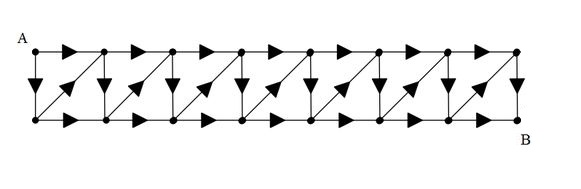# Some gridThe diagram above shows a tiling of 7 squares with $A$ and $B$ denoting the initial point and destination point, respectively. The arrow signs indicate the direction that you're able to travel to (east, south, north-east). Find the total number of ways to reach point $B$ from point $A$.

Bonus: Generalize this for $n$ squares.

×